# 5.7.4 The Motion of Vehicles in the Air (Structured Questions)

Question 1:
Diagram 1 shows an experiment to study Bernoulli’s Principle.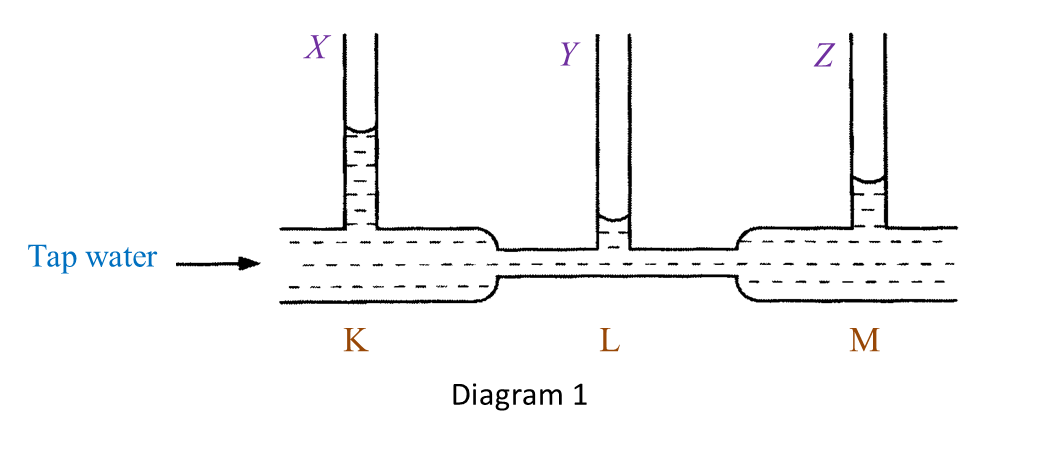(a)(i) State one observation of the water level in Diagram 1. [1 mark]

(ii) State one inference that can be made based on the observation in (a)(i). [1 mark]

(b) State the responding variable in this experiment. [1 mark]

(c) Based on this experiment, state the operational definition for Bernoulli’s Principle. [1 mark]

(d) Mark (\/) in the boxes provided to show the apparatus where the Bernoulli’s Principle is applied. [1 mark]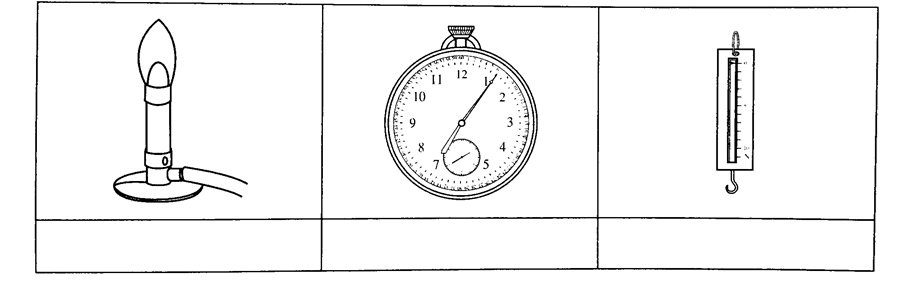(a)(i)
- The water level in the vertical tube Y is the lowest.
- The water level in the vertical tube X is the highest.
(any one)

(a)(ii)
- The water at the base of the vertical tube Y exerts the lowest pressure.
- The water at the base of the vertical tube X exerts the highest pressure.
(any one)

(b)
The pressure exerted by the moving water.

(c)
Bernoulli’s Principle states that pressure in a liquid decreases when the speed of the liquid increases.

(d)# 5.7.3 The Motion of Vehicles in Water (Structured Questions)

Question 1:
Diagram 1 shows an experiment to study the relationship between upthrust and the weight of water displaced.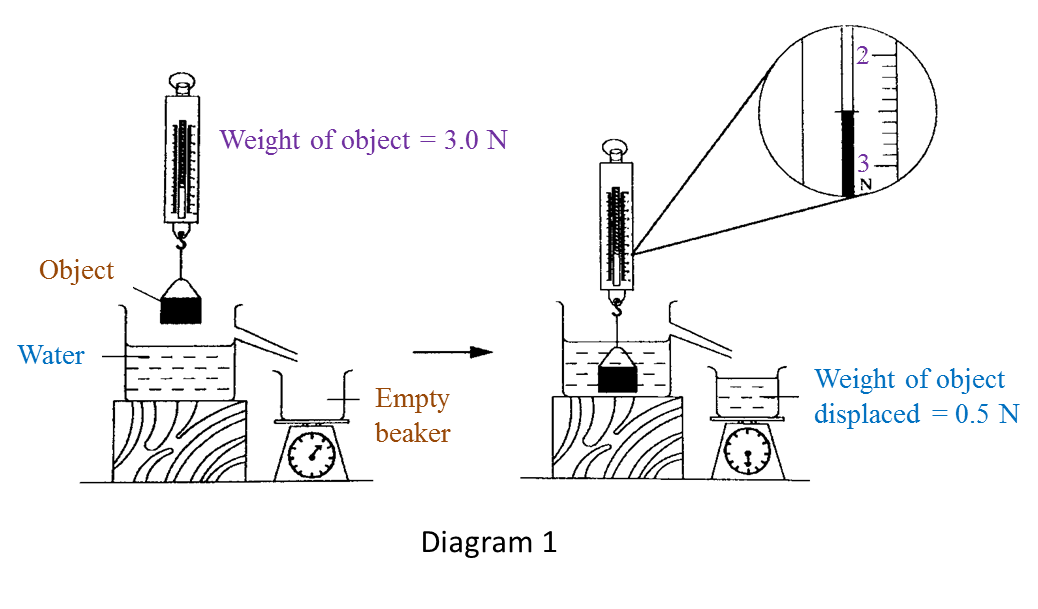(a) Based on Diagram 1, state the weight of object in water and write down your answer in the Table below. [1 mark]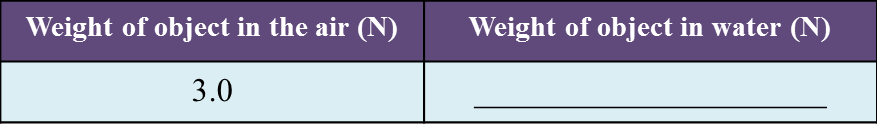(b) Observe Diagram 1. Compare the weight of object in the air and in water. [1 mark]

(c) State one inference for this experiment. [1 mark]

(d) State one responding variable in this experiment. [1 mark]

(e) The upthrust acts on an object when it is immersed in water.
State the operational definition of upthrust[1 mark]

:
(a)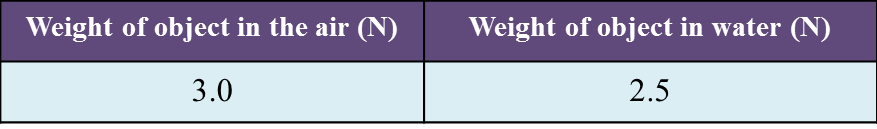(b)
The weight of object in the air is larger than the weight of object in the water.

(c)
The apparent loss in weight is equal to the weight of water displaced.

(d)
Weight of water displaced.

(e)
Upthrust is the apparent loss in weight of an object when it is immersed in water.

Question 2:
Diagram 2.1 shows the weight of a metal block in the air.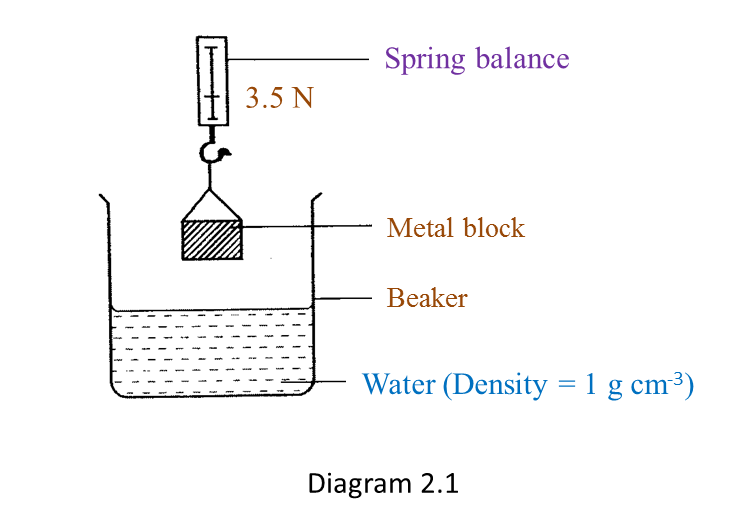Diagram 2.2 shows the apparent weight of the metal block when it is immersed in water.(a) Based on Diagram 2.1 and Diagram 2.2:
(i) State one observation from the result of this experiment. [1 mark]

(ii) State one inference from this experiment. [1 mark]

(b) State the constant variable in this experiment. [1 mark]

The experiment is repeated by using sea water as shown in Diagram 2.3.(c) Based on Diagram 2.3, what is the reading on the spring balance? [1 mark]

(d) Predict the reading of the spring balance if the metal block is immersed in a liquid which has higher density than sea water. [1 mark]

(a)(i)
The weight of the metal block in air is larger than the apparent weight of the same metal block immersed in water.

(a)(ii)
An upthrust acts on the metal block when it is immersed in water.

(b)
Metal block, water (density = 1 g cm-3). (any one)

(c)
2.4 N

(d)
The reading of the spring balance will be lower than the reading shown in Diagram 2.3.

# 5.7.2 The Concept of Pressure (Structured Questions)

Question 1:
Figure 1.1 shows an experiment to study the pressure produced by metal blocks, A and B of the same mass.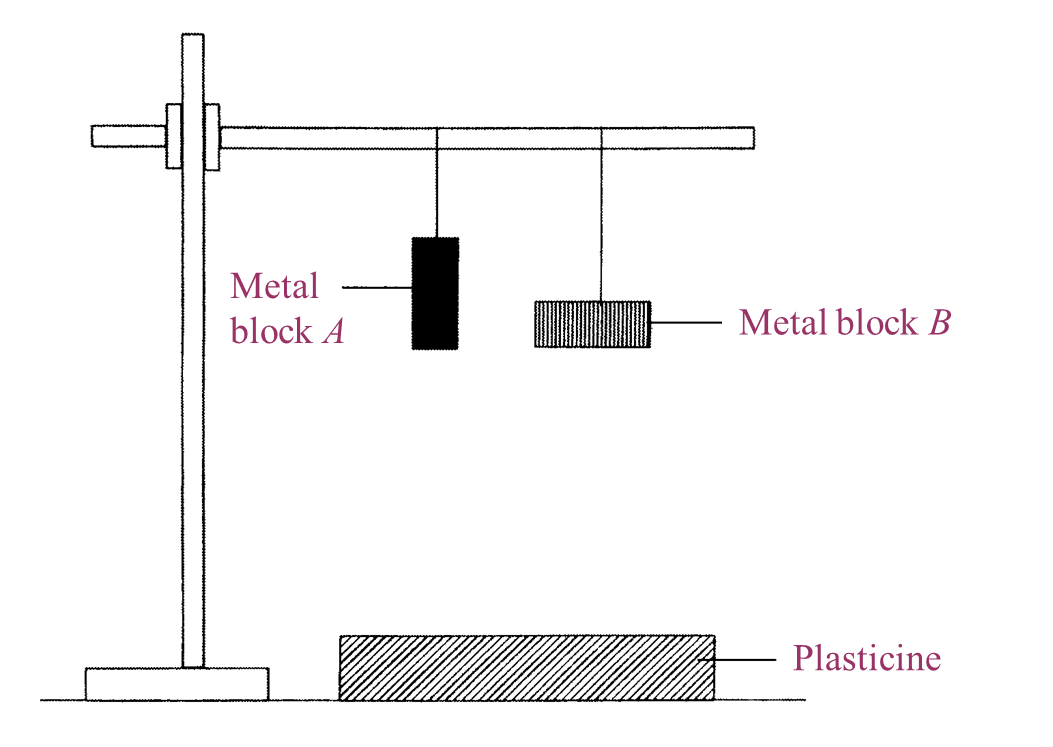Figure 1.1

Figure 1.2 shows the effect on the plasticine when metal blocks, A and B were dropped.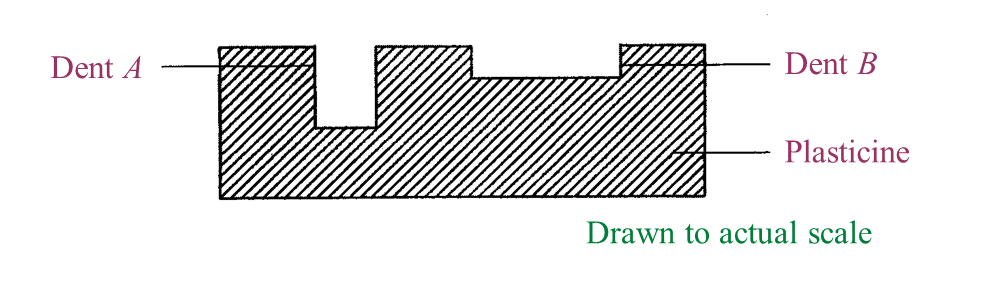Figure 1.2

(a)
What is the controlled variable in this experiment? [1 mark]

(b)
Write down one observation from the result of this experiment. [1 mark]

(c)
State one inference that can be made based on the observation in Figure 1.2. [1 mark]

(d)
Based on Figure 1.2, measure and record the depth of dent A. [1 mark]

(e)
Metal block C in Figure 1.3 has the same mass as metal blocks, A and B.Figure 1.3

Predict the depth of dent produced on the plasticine when metal block C is dropped from the same height. [1 mark]

(a)
The weight of the metal block (dropped from the same height).

(b)
The depth of dent A is more than the depth of dent B.

(c)
The surface area of A is smaller than the surface area of B.

(d)
The depth of dent A is 1 cm.

(e)
Deeper than dent A (or more than 1 cm).

# 5.7.1 The Concept of Momentum (Structured Questions)

Question 1:
Diagram 1.1 and Diagram 1.2 show an experiment to study the collision between trolleys of different masses with a plasticine block.Both trolleys move with the same velocity.
(a) State the variables in this experiment.
(i) Manipulated variable [1 mark]
(ii) Responding variable [1 mark]

(b)(i) What can you observe about the plasticine block after the collision? [1 mark]
(ii) State one inference that can be made based on the observation in (b)(i). [1 mark]

(c) State the hypothesis of this experiment. [1 mark]

(a)(i)
Mass of the trolley

(a)(ii)
Depth of the dent on the plasticine block

(b)(i)

Trolley T produced a deeper dent on the plasticine block compared to the dent produced by trolley S after their collision with the plasticine block.

(b)(ii)

When a moving trolley with a bigger mass collides with a plasticine block, it produces a deeper dent on the plasticine block.

(c)
The bigger the mass of a moving trolley which collides with a plasticine block, the deeper the dent which will be formed on the plasticine block.

Question 2:
Diagram 2.1 shows an experiment to study the collision of a trolley with block M. After collision, block M is displaced to a new position.
The experiment is repeated using two trolleys as shown in Diagram 2.2.The result of this experiment is shown in Table below.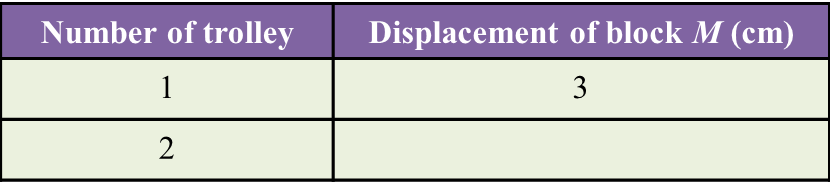(a) Measure the displacement of block M after the collision in Diagram 2.2.  [1 mark]

(b) State one observation about displacement of block M in diagram 2.1 and 2.2[1 mark]

(c) State the variables in this experiment.
(i) Constant variable [1 mark]
(ii) Manipulated variable [1 mark]

(d) Predict the displacement of block M if three trolleys are used. [1 mark]